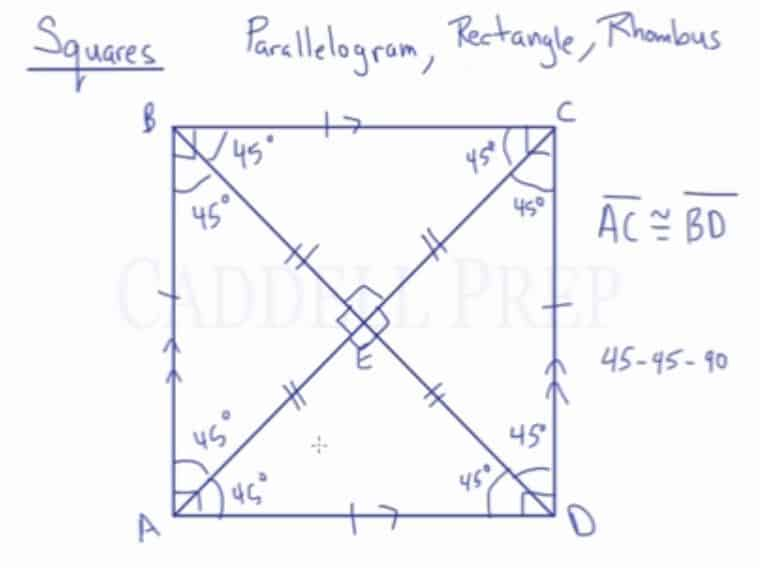Learn about the properties of squares including relationships among opposite sides, opposite angles, adjacent angles, diagonals and angles formed by diagonals.

Square: A quadrilateral with four congruent sides and four right angles.

Squares are special types of parallelograms, rectangles, and rhombuses. It has properties of all three, yet also has its own unique features.

All the sides in a square are congruent. Opposite sides are parallel.

All angles are congruent because of they all measure$90^\circ$.

The diagonals are congruent. They are perpendicular bisectors to each other. All of the line segments formed are congruent. The diagonals also bisect the angles at each vertex. They are all$45\textdegree$. This forms four isosceles right triangles with angle measures 45-45-90.## Video-Lesson Transcript

Here, we have a square$ABCD$.

A square is a special type of parallelogram.

It’s also a special type of rectangle. It’s a rectangle where all four sides are the same.

And it’s a special type of rhombus. It’s a rhombus with four right angles.

It’s a parallelogram because opposite sides are parallel. And opposite sides are also congruent.

It’s a rectangle because it’s a parallelogram that has four right angles.

And it’s a rhombus because it’s a parallelogram where all four sides are congruent.

Now, let’s see what happens when we draw diagonals.

Diagonal$AC$ and diagonal$BD$ are congruent, just like a rectangle.$\overline{\rm AC} \cong \overline{\rm BD}$

And all four of these line segments are congruent, just like in a rectangle.

Just like a rhombus, when these diagonals intersect, they form right angles. So, we have four right triangles.

And, just like in a rhombus, the diagonals bisect these angles. So, these angles are congruent and all four of these are the same.

What’s special with a square is that all of these angles that are bisected are going to be the same.

They’re all$45^{\circ}$.

So, we end up with four triangles have angles$45 - 45 - 90$. These are four isosceles right triangles.